# CALCULUS

Prof.  G. Battaly, Westchester Community College

Schedule          Class Notes         Flash cards        Homework

Homework Help:                       Step-by-Step Procedures
Powerpoint Lessions
Problems:

Prerequisites Chapter | Chapter 1 | Chapter 2 | Chapter 3Chapter 4 |

| Chapter 5 | Chapter 6 | Chapter 7 | Chapter 8 |

#### Chapter 5 Ch 5.1:  The Natural Logarithm and Differentiation:   Prob. 31-47, 53-63 Ch 5.2:  The Natural Logarithm and Integration:   Prob. 1-21, 23-37 Ch 5.3:  Inverse Functions:   Prob. 1-41, 43-67 Ch 5.4:  Exponential Functions: Differentiation and Integration   Prob. 27-51, 53-73 Ch 5.6:  Inverse Trig Functions and Differentiation Ch 5.7:  Inverse Trig Functions and Integration

Chapter 6
Ch 6.1
Differential Equations & Slope Fields

Ch 6.2
Differential Equations:  Growth & Decay
Ch 6.3
Differential Equations:  Separation of Variables

Ch 6.4
First Order Differential Equations  Prob.  1 - 9

#### Chapter 8 Ch 8.1:   Basic Integration Ch 8.2:  Integration by Parts   Prob. 1-17, 19-27, 29-33 Ch 8.3:   Integrals with Trig Ch 8.4:  Trigonometric Substitution   Prob. 1-23, 27-45 Ch 8.5:  Partial Fractions   Prob. 1-15, 17-23 Ch 8.7:  Indeterminate Forms & L'Hopital's Rule   Prob. 1-23, 31-37 Ch 8.8:   Improper Integrals

Prequisites Chapter - see below   This may be of benefit to students who need additional work.
Ch P.1Real Numbers:  Problems 11-35Problems 39-59
Ch P.2The Cartesian Plane:  Problems 17-37Problems 39-53
, Problems 55-59
Ch P.3Graphs of Equations:  Problems 17-29 Problems 53-69
Ch P.4Lines in the Plane:  Problems 13-39Problems 41-55
, Problems 57-67
Ch P.5
Functions:  Problems 5-35Problems 41-59
Ch P.6
Trigonometry:  Problems 9-31Problems 33-55
,
Problems 57-87

Homework Help:  Step-by-Step Procedures
Word Problems     Related Rates
Find Absolute Extrema on a Closed Interval [a,b]
Relative Extrema, Increasing & Decreasing Functions, & the First Derivative Test

**
Finding Relative Extrema given f '(x) (a gif animation) **
Relative Extrema, Concavity and the Second Derivative Test
Limits at Infinity for Rational Functions
Curve Sketching
Optimization Problems
Using the Limit Definition to Find Area
Integrating Rational Expressions

Solving a First Order Linear Differential Equation
Finding the Area of the Region Bounded by 2 or more Curves
Volumes of Revolution - Disk Method

Volumes of Revolution - Which Method?

Volumes of Revolution - Shell Method

How to Do Word Problems:

2. Read the problem again, identifying what's given and what you need to find.
3. Organize the information:  draw a diagram, construct a table, etc.
4. Identify the unknown variables, including the appropriate rates, such as dy/dt or dA/dt, etc.
5. Write an equation to relate the given and the to find.
6. Solve the equation.  For related rates, this involves implicit differentiation.
7. Check the solution:   Is "to find" found?
Does solution make sense?
Do numbers fit?

RELATED RATES:

1. Identify: a "given" rate, a "to find" rate, other conditions
2. Determine the relationship (equation) between the given and the to find. Use a diagram, if possible, or know formula.
3. If possible, use the given information to reduce the number of variables.
4. Differentiate implicitly with respect to time. This always involves the chain rule. For example,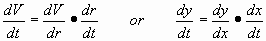5. Substitute the given values and solve for the unknown rate.
6. Check:
• Did you include units?
• Does the answer make sense?
• If there were angles involved, did you use radians?

1. Is the denominator a monomial?
If yes, divide and integrate.
If no, proceed to #2.
2. If the quotient is rewritten as a product, is the exponent (-1)?
If yes, think   ln   and look for   du/u
If no, think   un   and look for   un du

1. Find relative extrema.
a)  Find critical numbers [f '(c) = 0 or f '(c) undefined]
b)  Find f(c) for all c.
2. Evaulate function at endpoints:  find f(a) and f(b)
3. Compare f values of relative extrema and endpoints and select:
a)  the point (x,f(x)) with the largest f value is the absolute maximum
b)  the point (x,f(x)) with the smallest f value is the absolute minimum

1. Find critical numbers [f '(c) = 0 or f '(c) undefined]
2. Determine intervals for evaluation of f ' and begin the interval table:
a)  Locate the critical numbers along a number line containing the domain of the function.
b)  Determine the intervals, using the critical numbers as endpoints.
3. Continue the interval table by:

a)  Selecting a test value for each interval.
b)  Express f '(x) in factored form, and write each factor in the first column.
c)  Find the sign of each factor in each interval and indicate the sign on the table.
d)  For each interval, find the sign of f '(x) by determining the number of negative factors.
4. Determine whether f(x), the original function, is increasing (when f '(x) >0) or decreasing (when f '(x) <0) on each interval.
5. The critical value for which f(x) is increasing to the left and decreasing to the right is a relative max.  /    \
The critical value for which f(x) is decreasing to the left and increasing to the right is a relative min.  \    /
6. Find the corresponding f or y value for each critical value determined to be a relative max or min, and write the ordered pair (c,f(c)).
1. Find critical numbers [f '(c) = 0 or f '(c) undefined]
2. Find f ''(x).
3. Find f ''(c) for all critical numbers.
4. Determine the relative extrema using the Second Derivative Test:
a)  If f ''(c) > 0, then f is concave up and f(c) is a relative min
b)  If f ''(c) < 0, then f is concave down and f(c) is a relative max
c)  If f ''(c) = 0, then the test fails.  (consider an Inflection Point - a point where concavity changes)

1. If degree of numerator < degree of denominator, then limit is 0.
2. If degree of numerator = degree of denominator, then limit is ratio of leading coefficients.
3. If degree of numerator > degree of denominator, then limit does not exist.
4. For the first 2 cases, where a limit k exists, then y = k is a horizontal asymptote.  Be sure to consider the limit approaching both positive infinity and negative infinity.

How to Do Optimization Problems:

1.  Read the problem carefully, and identify what's given and what you need to find.
2.  Organize the info: draw a diagram, construct a table, etc.
3.  Identify the unknown variables; add to diagram or table.
4.  Write an equation to relate the given and the to find.
5.  Reduce the number of variables to 2.
6.  Find the derivative and Critical Numbers.
7.  Test the critical numbers for max or min, using 1st derivative or 2nd derivative test, and state solution.
8.  Check the solution: Is "to find" found? Does solution make sense? Do numbers fit?

1. Sketch the function f and indicate the region on the interval [a,b].
2. Is f continuous and nonnegative on the interval?  If yes, then: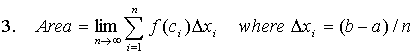5.   Substitute ci for x in the function and proceed with the sums, using Summation Formulas, Theorum 4.2.
6.   Evaluate the limits at infinity.

Curve Sketching:        DRIve A Car NEXt TRIP

D:  Domain,        R:  Range,        I:  Intercepts,       A:  Asymptotes,
CN:  Critical Numbers,         EXTR:  Extrema,         IP:  Inflection Points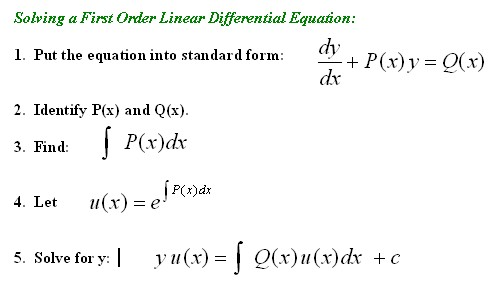1. Sketch the curves:
• Find the points of intersection of the curves.
• If the curves are close and orientation is difficult to determine, substitute values between those of the points of intersection to determine which is above (or to the right of) the other.
2. Use the sketch to determine which integral to use:
• If each curve passes the vertical line test in the bounded region, use vertical rectangles, the x variable, and the integral: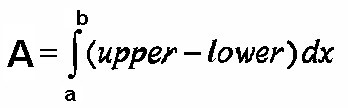• If a curve fails the vertical line test but passes the horizontal line test in the bounded region, use horizontal rectangles, the variable y, and the integral: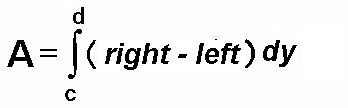3. If the bounded area contains more than one distinct region, write the area as the sum of the areas of each distinct region.

4. Limits of integration:
• Use the coordinates of the points of intersection.
• If x = k1  or y = k2  is given this may be one of the limits.

Volumes of Revolution - Disk Method

1. Sketch the curves and identify the region, using the points of intersection.
2. Locate the axis of revolution on the sketch.
3. Decide whether to use a horizontal or vertical rectangle.  The rectangle should be perpendicular to the axis of revolution.
4. Sketch the rectangle and determine the variable of integration.

a)  If the rectangle is horizontal, then integrate with respect to y (use dy).  The integrand must be in terms of y.
b)  If the rectangle is vertical, then integrate with respect to x (use dx).  The integrand must be in terms of x.
5. Determine the integrand:      R2,    or    R2 - r2    ?
a)  If the rectangle touches the axis of revolution, identify R as the length of the rectangle.  Find R in terms of the appropriate variable (see above), and use R2 as the integrand.
b)  If the rectangle does not touch the axis of revolution, identify R as the distance of the furthest end of the rectangle from the axis of revolution and r as the distance of the closest end of the rectangle from the axis of revolution.  Use  R2 - r2  as the integrand.

1. Sketch the curves and identify the region, using the points of intersection.
2. Locate the axis of revolution on the sketch.
3. Decide whether to use a horizontal or vertical rectangle.  Select the orientation that requires the least number of separate sections.
4. Decide whether to use the Disc Method or the Shell Method:

a)  If the rectange is perpendicular to the axis of revolution, use the Disc Method.
b)  If the rectangle is parallel to the axis of revolution, use the Shell Method.

Volumes of Revolution - Shell Method

1. Complete Steps 1 to 4 in Volumes of Revolution, which Method? noted above.
2. Be sure that your rectangle is parallel to the axis of revolution.
3. Determine the variable of integration:
a)  If the rectangle is horizontal, then integrate with respect to y (use dy).  The integrand must be in terms of y.
b)  If the rectangle is vertical, then integrate with respect to x (use dx).  The integrand must be in terms of x.
4. Determine the integrand:    p(x)h(x) or p(y)h(y) ?

 a)  If the rectangle is horizontal, identify p(y), the distance of the rectangle from the axis of revolution, and h(y), the length of the rectangle.  Use: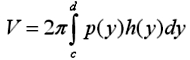b)   If the rectangle is vertical, identify p(x), the distance of the rectangle from the axis of revolution, and h(x), the length of the rectangle.  Use:Webmaster:  G. Battaly, merlin@pipeline.com
Last updated:
January 13, 2016